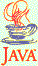### Arithmetic Evaluator

14 points

Implement the following algorithm for the evaluation of arithmetic expressions.

Each operator has a precedence.  The + and - operators have the lowest (and equal) precedence, * and / have a higher (and equal) precedence, and ^ (which denotes "raising to a power" in this exercise) has the highest.  For example,

3 * 4 ^ 2 + 5

should mean the same as

(3 * (4 ^ 2)) + 5

with a value of 53.

In your algorithm, use two stacks.  One stack holds numbers, the other holds operators.

Here is how the expression 3 * 4 ^ 2 + 5 is evaluated:

 Expression: 3 * 4 ^ 2 + 5 1 Remaining expression: * 4 ^ 2 + 5 Number stack 3 Operator stack 2 Remaining expression: 4 ^ 2 + 5 Number stack 3 Operator stack * 3 Remaining expression: ^ 2 + 5 Number stack 4 3 Operator stack * 4 Remaining expression: 2 + 5 Number stack 4 3 Operator stack ^ * 5 Remaining expression: + 5 Number stack 2 4 3 Operator stack ^ * 6 Remaining expression: + 5 Number stack 16 3 Operator stack * 7 Remaining expression: 5 Number stack 48 Operator stack + 8 Remaining expression: Number stack 5 48 Operator stack + 9 Remaining expression: Number stack 53 Operator stack

The program does not handle negative numbers. It cannot distinguish between "-" as an operator, and the "-" that makes a number negative.

This is Project 15.3 from p. 696 of Java Concepts for AP Computer Science.

Test your program by running these test cases:

 # Test Case Result 1 9 - 6 / 3 7 2 8 - 6 + 3 5 3 9 + 3 * 2 - 8 / 4 13 4 4 * 3 - 6 / 2 9 5 9 / 3 * 2 / 4 * 2 3 6 7 - 6 + 5 - 4 + 3 5 7 8 + 4 - 3 / 3 * 2 10

### Extra Credit #1

3 points

Enable your program to work with numbers other than the integers from 0 to 9. Some guidance on this is given in Arithmetic.java.

Test your program by running these test cases:

 # Test Case Result 1 12 / 3 - 10 * 2 -16 2 15 - 12 + 9 - 6 + 3 9 3 12 + 10 / 5 - 12 / 6 12 4 12 + 24 / 3 / 4 - 3 11 5 15 - 2 * 11 * 3 + 17 -34

### Extra Credit #2

4 points

Enable your program to handle parentheses. Some guidance on this is given in Arithmetic.java.

Test your program by running these test cases:

 # Test Case Result 1 (4 + 6 - 2) / (7 - 3 + 4) 1 2 9 - (3 + 4) / 7 8 3 9 - (3 + 4) - (2 - 5) 5 4 (6 - 2) * 3 - 2 * (3 - 5) 16 5 (6 + 2) / 4 - 9 / (2 + 1) -1

If you did both extra-credits, test your program by running these test cases:

 # Test Case Result 1 (322 - 111 - 43) / (21 - 4 - 3) 12 2 132 - (21 + 15) / 12 129 3 270 / (9 * 5) / 2 + (42 + 43) * 12 * 2 2043 4 (119 + 1) / (21 - 13) - (211 - 36) / (19 + 6) 8 5 (53 + 45) / 7 + (6 - 4) * 2 18

Documentation for this program is available here.

Starting Point

You can download Arithmetic.java to use as a starting point in writing your program. Instructions for writing the program are given in the .java file. You'll also need the Stack interface (Stack.java) and the ArrayStack.java file you wrote for a previous program.

### What Does the Program Do?

You can download Arithmetic.jar, which will show you how this program can function when you've completed it. Double-click on the JAR file to get the program to run.